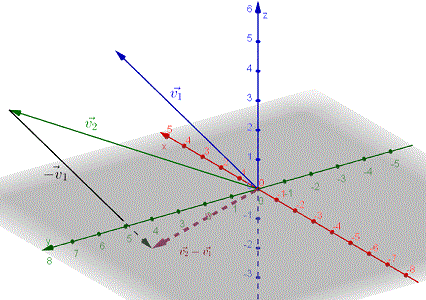# 3D Vectors (R3)

3D vectors are introduced along with oprations such the sum, difference and scalar multiplication. Also the properties such as the magnitude are included. Questions with detailed solutions are included.

What is a Vector?

A vector is a quantity that has both a magnitude and a direction. It is geometrically represented by a line segment whose length is the magnitude and an arrow that indicates its direction as shown in the figure below. Vectors are be used in physics to model quantities with sizes and directions such as velocities, forces, accelerations; in engineering, chemistry, computer graphics, robotics, and many other fields.In the above, the vector is defined using an initial point A and a terminal point B. Therefore the vector may be denoted as $\vec{AB}$.

Equivalent Vectors

Vectors with equal magnitudes and same direction are equivalent vectors.Sum of two Vectors

Given two vectors $\vec{v_1}$ and $\vec{v_2}$, their sum is a vector obtained by first positioning vector $\vec{v_2}$ such that its initial point coincide with the terminal point of $\vec{v_1}$ and the sum $\vec{v_1} + \vec{v_2}$ is the vector whose initial point is the initial point of $\vec{v_1}$ and its terminal point is the terminal point of $\vec{v_2}$. Note that $\vec{v_1} + \vec{v_2} = \vec{v_2} + \vec{v_1}$. Also the sum of two vectors coincide with the diagonal of the parallelogram determined by $\vec{v_1}$ and $\vec{v_2}$.Difference of two Vectors

Given two vectors $\vec{v_1}$ and $\vec{v_2}$, the difference $\vec{v_2} - \vec{v_1}$ may be defined as a sum $\vec{v_2} + (- \vec{v_1})$ and represented geometrically as shown below.Multiplication of a Vector by a Scalar

A vector $\vec{v_1}$ multiplied by a scalar $k$ is defined as a vector $k\vec{v_1}$ parallel to $\vec{v_1}$ and whose direction is the same as that of $\vec{v_1}$ if k > 0 and opposite if k <0 . The magnitude (length) of $k\vec{v_1}$ is $| k |$ times the magnitude of $\vec{v_1}$. Figure below shows vectors $\vec{v_1}$, $2\vec{v_1}$ and $-3\vec{v_1}$.Vectors in a 3D Rectangular Coordinate System

A unit vector is a vector with magnitude equal to 1. Below is a shown a 3D rectangular coordinate system with unit vectors $\vec{i}$, $\vec{j}$ and $\vec{k}$ in the positive direction of the x, y and z axes respectively. Vectors $\vec{i}$, $\vec{j}$ and $\vec{k}$ may be defined by their components as follows:
$\vec{i}$ = <1,0,0> , one unit along the x axis.
$\vec{j}$ = <0,1,0> , one unit along the y axis.
$\vec{k}$ = <0,0,1> , one unit along the z axis.Components of a Vector

The components of any vetor $\vec{v}$ are defined by expressing $\vec{v}$ as a sum of multiples of the unit vectors $\vec{i}$, $\vec{j}$ and $\vec{k}$ as follows:
$\vec{v} = 3\vec{i} + 4\vec{j} + 5\vec{k}$
or in components form as follows:
$\vec{v} = <3,4,5>$The components of a vector $\vec{v}$ defined by its initial point $A = (x_1 , y_1 ,z_1)$ and its terminal point $B = (x_2 , y_2 ,z_2)$ are given by
$\vec{v} = < x_2-x_1,y_2-y_1,z_2-z_1>$Use Components of a Vector to Calculate its Magnitude and Unit vector in the Same Direction

Given vector $\vec{v} = < a,b,c>$, its magnitude (or length) is given by
$||\vec{v}|| = \sqrt{a^2+b^2+c^2}$.
The
unit $\vec{u}$ vector, defined as a vector of magnitude equal to 1, in the same direction as $\vec{v}$ is given by
$u = \dfrac{1}{||\vec{v}||} \vec{v}$

Use Components of Vectors to Calculate the Sum, Difference, and Scalar multiplication of Vectors

Given vectors $\vec{v_1} = < a_1,b_1,c_1>$ and $\vec{v_2} = < a_2,b_2,c_2>$, the sum $\vec{v_1} + \vec{v_2}$ , the difference $\vec{v_1} - \vec{v_2}$ and scalar multiplication $k \vec{v_1}$, k a real number, are given by
$\vec{v_1} + \vec{v_2} = < a_1+a_2,b_1+b_2,c_1+c_2>$
$\vec{v_1} - \vec{v_2} = < a_1 - a_2,b_1 - b_2,c_1 - c_2>$
$k \vec{v_1} = < k a_1,k b_1,k c_1>$

Questions on 3D Vectors.

Detailed
Solutions and explanations to the questions below are included.
1) Find the components of the vectors $\vec{AB}$ and $\vec{BA}$ where A and B are points given by their coordinates A(2,6,7) and B(0,-3,1) and show that $\vec{AB} = -1 \vec{BA}$.
2) Given vectors $\vec{v_1} = <0,-3,2>$ and $\vec{v_2} = <-3,4,5>$, find:
a) $\vec{v_1} + \vec{v_2}$
b) $\vec{v_1} - \vec{v_2}$
c) $-3\vec{v_1}$
d) $-2\vec{v_1} + 3\vec{v_2}$
e) $k$such that $||\vec{v_1} + k\vec{v_2}|| = \sqrt{67}$.
3) Given vector $\vec{v} = <0,-3,2>$, find the unit vector in the same direction as $\vec{v}$ and check that its magnitude is equal to 1.
4) Given the points A(2,6,7), B(0,-3,1) and C(0,3,4), find the components of the vectors $\vec{AB}$, $\vec{AC}$ and $\vec{BC}$ and show that $\vec{AB} + \vec{BC} = \vec{AC}$.
5) Given the points A(-1,2,1), B(2,4,2) and C(5,6,3), find the components of the vectors $\vec{AB}$, $\vec{BC}$ and $\vec{AC}$ and determine which of these vectors are equivalent and which are parallel.

6) Given vectors $\vec{v_1} = <-4,0,2>$ and $\vec{v_2} = <-1,-4,2>$, find vector $\vec{v}$ such that $\vec{v_1} - 2 \vec{v} = 3 \vec{v} - 3 \vec{v_2}$
7) Find a vector in the same direction as vector $\vec{v} = <-4,2,2>$ but with twice the length of $\vec{v}$.
8) Find a vector in the opposite direction of vector $\vec{v} = <-1,2,2>$ but with a length of 5 units.
9) Given vector $\vec{v} = <-1,2,2>$, find a real number $k$ such that $||k \vec{v} || = 1/5$.
10) Find $b$ and $c$ such that vectors $\vec{v_1} = <-4,6,2>$ and $\vec{v_2} = <2,b,c>$ are parallel.
11) Are the three points A(2,6,7), B(1,4,5) and C(0,2,3) collinear?
12) A cube of side 2 units is shown below.
a) Find the components of the vectors $\vec{AB}$, $\vec{EF}$, $\vec{DC}$, $\vec{HG}$, $\vec{AC}$ and $\vec{AG}$.
b) Which of the vectors in part a) are equivalent?
c) Prove algebraically that $\vec{AB} + \vec{BF} + \vec{FG} = \vec{AC} + \vec{CG}$.
d) Find $|| \vec{AG} ||$.
e) Find the unit vector in the same direction as vector $\vec{AG}$.Detailed Solutions and explanations to these questions.